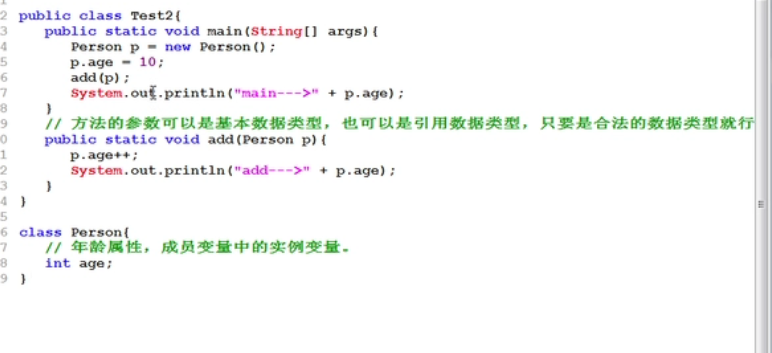# 成员方法的传参机制``````public class MethodParameter01 {

//编写一个 main 方法
public static void main(String[] args) {

int a = 10;
int b = 20;
//创建 AA 对象 名字 obj
AA obj = new AA();
obj.swap(a, b); //调用 swap

System.out.println("main 方法 a=" + a + " b=" + b);//a=10 b=20
}
}
class AA {
public void swap(int a,int b){
System.out.println("\na 和 b 交换前的值\na=" + a + "\tb=" + b);//a=10 b=20
//完成了 a 和 b 的交换
int tmp = a;
a = b;
b = tmp;
System.out.println("\na 和 b 交换后的值\na=" + a + "\tb=" + b);//a=20 b=10
}
}``````-- 展开阅读全文 --

« 上一篇 07-24